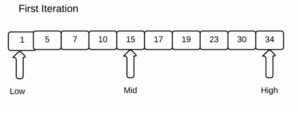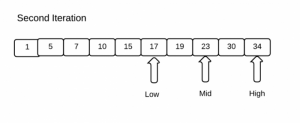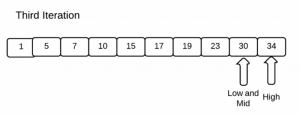There are two basic searching algorithms used. One is the simplest technique and is discussed in the below link.

The other search method is the binary search method. The binary search technique is a very fast and a more efficient technique when compared to the linear search method. The only requirement for this method is that the input array of elements must be in the sorted order.

In binary search, the target value (the element that has to be searched) is searched with the center element in the array. If the target value and the center element is equal, searching is complete, if it is not equal then the sample space is split into two parts, the element group on the left of the center element (values lower than the center element) or the element group on the right of the center element (values larger than the center element). As the elements are in the sorted order, the search process will now be restricted to either of the two element groups. As the process is repeated, the target value is found eventually.

The binary search process is explained below with the help of figures. Consider the sample space of 10 elements 1, 5, 7 ,10, 15, 17, 19, 23, 30 and 34 where the element 30 is the target value that has to be found.

In the beginning of the search process, 1 is the low value and 34 is the high value, the middle value is found using the formula (low+high)/2 and thus 15 is the center element or the middle element.Now as the target value 30 is greater than the center value 15, the search process in the next iteration will be restricted to the right half of the sample space i.e. from 17 to 34. For the second iteration, 17 and 34 are the low and high values respectively and 23 is the center value.The target value is compared with the present center value i.e. 23. So the search process will be restricted to the elements on the right to the center element 23. So the low value is 30 and the high value is 34. Now, the center element is 30. In the third iteration, the target value equals the center element 30 and thus the searching process is complete.The code for binary search is given below

```#include<stdio.h>
int main()
{

/* Here we initialize the value of the sample space in an array named ss*/
int ss={1,5,7,10,15,17,19,23,30,34};
int low=0, high=9, mid,tar;

/* The target value is to be taken as input */
printf("\nEnter the target value");
scanf("%d",&tar);

/* Value of mid is the average of low and high*/
for(mid=(low+high)/2;low<high;mid=(low+high)/2)
{
/* The middle value in the array is compared with the target */
if(ss[mid]==tar)
{
printf("\nThe target value has been found at position %d",mid+1);
break;
}

/* If the target value is less than the present middle value, then
target is present between low and present middle, so high is altered */
else if(ss[mid]>tar)
high=mid-1;

/* If the target value is greater than the present middle value, then
target is present between present middle and high, so low is altered */
else
low=mid+1;
}
return 0;
}```
Share.